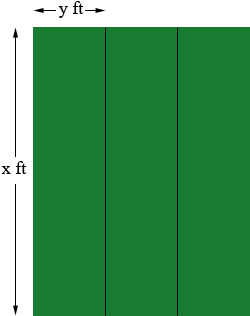SEARCH HOMEMath Central Quandaries & QueriesQuestion from Jean, a teacher: An animal clinic wants to construct a kennel with 3 individual pens, each with a gate 4 feet wide and an area of 90 square feet. The fencing does not include the gates. Write a function to express the fencing as a function of x. Find the dimensions for each pen, to the nearest tenth of a foot that would produce the required area of 90 square feet but would use the least fencing. What is the minimum fencing to the nearest tenth?Hi Jean,

I expect that you are to consider rectangular pens. If you want to minimize the amount of fencing required then you want pens to share sides as much as possible so my diagram is below.I let x feet be the length of each pen in one direction and y feet be the length in the other dimension. Since the area of each pen is 90 square feet

x × y = 90 square feet.                                       (1)

The fencing required is 4 pieces of length x feet each and 6 pieces of length y feet each minus 3 × 4 = 12 feet for the gates. Thus the length L of fencing requited is

L = 4x + 6y - 12 feet.                                       (2)

Solve equation (1) for y and substitute into equation (2). This will give you the function you need to minimize.

I hope this helps,
HarleyMath Central is supported by the University of Regina and The Pacific Institute for the Mathematical Sciences.